# Linear 100x100 Work-Precision Diagrams

##### Chris Rackauckas

For these tests we will solve an 100x100 system of linear differential equations. This will demonstrate the efficiency of the methods for handling large systems. We will be mostly looking at the efficiency of the work-horse Dormand-Prince Order 4/5 Pairs: one from DifferentialEquations.jl (DP5, one from ODE.jl rk45, and one from ODEInterface: Hairer's famous dopri5).

Also included is Tsit5. While all other ODE programs have gone with the traditional choice of using the Dormand-Prince 4/5 pair as the default, DifferentialEquations.jl uses Tsit5 as one of the default algorithms. It's a very new (2011) and not widely known, but the theory and the implimentation shows it's more efficient than DP5. Thus we include it just to show off how re-designing a library from the ground up in a language for rapid code and rapid development has its advantages.

## Setup

using OrdinaryDiffEq, Sundials, DiffEqDevTools, Plots, ODEInterfaceDiffEq, ODE
using Random
Random.seed!(123)
gr()
# 2D Linear ODE
function f(du,u,p,t)
@inbounds for i in eachindex(u)
du[i] = 1.01*u[i]
end
end
function f_analytic(u₀,p,t)
u₀*exp(1.01*t)
end
tspan = (0.0,10.0)
prob = ODEProblem(ODEFunction(f,analytic=f_analytic),rand(100,100),tspan)

abstols = 1.0 ./ 10.0 .^ (3:13)
reltols = 1.0 ./ 10.0 .^ (0:10);


### Speed Baseline

First a baseline. These are all testing the same Dormand-Prince order 5/4 algorithm of each package. While all the same Runge-Kutta tableau, they exhibit different behavior due to different choices of adaptive timestepping algorithms and tuning. First we will test with all extra saving features are turned off to put DifferentialEquations.jl in "speed mode".

setups = [Dict(:alg=>DP5())
Dict(:alg=>ode45())
Dict(:alg=>dopri5())
Dict(:alg=>ARKODE(Sundials.Explicit(),etable=Sundials.DORMAND_PRINCE_7_4_5))
Dict(:alg=>Tsit5())]
solnames = ["OrdinaryDiffEq";"ODE";"ODEInterface";"Sundials ARKODE";"OrdinaryDiffEq Tsit5"]
wp = WorkPrecisionSet(prob,abstols,reltols,setups;names=solnames,save_everystep=false,numruns=100)
plot(wp)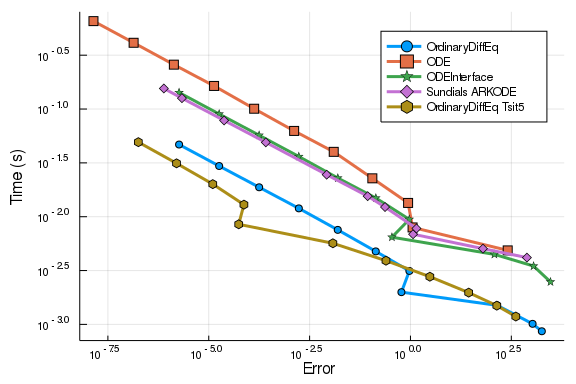OrdinaryDiffEq.jl is clearly far in the lead, being more than an order of magnitude faster for the same amount of error.

### Full Saving

setups = [Dict(:alg=>DP5(),:dense=>false)
Dict(:alg=>ode45(),:dense=>false)
Dict(:alg=>dopri5()) # dense=false by default: no nonlinear interpolation
Dict(:alg=>ARKODE(Sundials.Explicit(),etable=Sundials.DORMAND_PRINCE_7_4_5),:dense=>false)
Dict(:alg=>Tsit5(),:dense=>false)]
solnames = ["OrdinaryDiffEq";"ODE";"ODEInterface";"Sundials ARKODE";"OrdinaryDiffEq Tsit5"]
wp = WorkPrecisionSet(prob,abstols,reltols,setups;names=solnames,numruns=100)
plot(wp)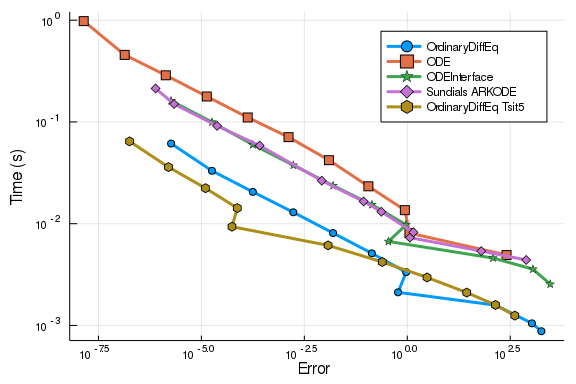While not as dramatic as before, DifferentialEquations.jl is still far in the lead. Since the times are log scaled, this comes out to be almost a 5x lead over ODEInterface, and about a 10x lead over ODE.jl at default tolerances.

### Continuous Output

Now we include continuous output. This has a large overhead because at every timepoint the matrix of rates k has to be deep copied.

setups = [Dict(:alg=>DP5())
Dict(:alg=>ode45())
Dict(:alg=>dopri5())
Dict(:alg=>ARKODE(Sundials.Explicit(),etable=Sundials.DORMAND_PRINCE_7_4_5))
Dict(:alg=>Tsit5())]
solnames = ["OrdinaryDiffEq";"ODE";"ODEInterface";"Sundials ARKODE";"OrdinaryDiffEq Tsit5"]
wp = WorkPrecisionSet(prob,abstols,reltols,setups;names=solnames,numruns=100)
plot(wp)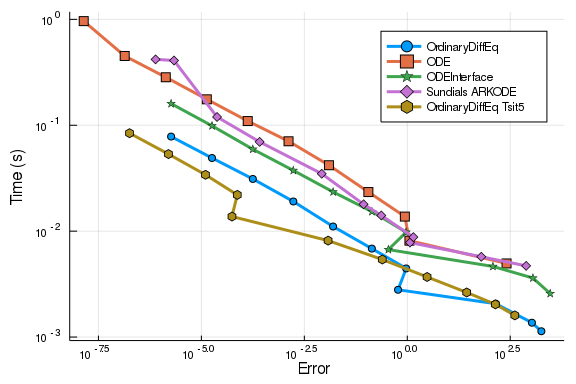As you can see, even with this large overhead, DifferentialEquations.jl essentially ties with ODEInterface. This shows that the fully featured DP5 solver holds its own with even the classic "great" methods.

### Other Runge-Kutta Algorithms

Now let's test it against a smattering of other Runge-Kutta algorithms. First we will test it with all overheads off. Let's do the Order 5 (and the 2/3 pair) algorithms:

setups = [Dict(:alg=>DP5())
Dict(:alg=>BS3())
Dict(:alg=>BS5())
Dict(:alg=>Tsit5())]
wp = WorkPrecisionSet(prob,abstols,reltols,setups;save_everystep=false,numruns=100)
plot(wp)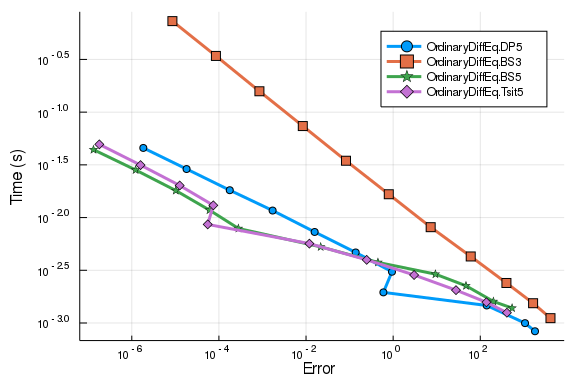As you can see, the Tsit5 algorithm is the most efficient, beating DP5 which is more efficient than the Bogacki-Shampine algorithms. However, you can see that when the tolerance is high, BS3 could be of use since its slope is so steep.

## Higher Order

Now let's see how OrdinaryDiffEq.jl fairs with some higher order algorithms:

setups = [Dict(:alg=>DP5())
Dict(:alg=>Vern6())
Dict(:alg=>TanYam7())
Dict(:alg=>Vern7())
Dict(:alg=>Vern8())
Dict(:alg=>DP8())
Dict(:alg=>Vern9())]
wp = WorkPrecisionSet(prob,abstols,reltols,setups;save_everystep=false,numruns=100)
plot(wp)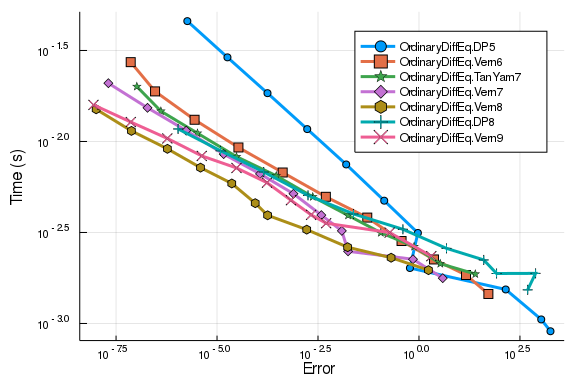Vern7 looks to be the winner here, with DP5 doing well at higher tolerances but trailing of when it gets lower as one would expect with lower order algorithms. Some of the higher order methods, such as Vern9, would do better at lower tolerances than what's tested (outside of floating point range).

## Higher Order With Many Packages

Now we test OrdinaryDiffEq against the high order methods of the other packages:

using LSODA
setups = [Dict(:alg=>DP5())
Dict(:alg=>Vern7())
Dict(:alg=>dop853())
Dict(:alg=>ode78())
Dict(:alg=>odex())
#Dict(:alg=>lsoda())
Dict(:alg=>ddeabm())
Dict(:alg=>ARKODE(Sundials.Explicit(),order=8))
Dict(:alg=>CVODE_Adams())]
wp = WorkPrecisionSet(prob,abstols,reltols,setups;save_everystep=false,numruns=100)
plot(wp)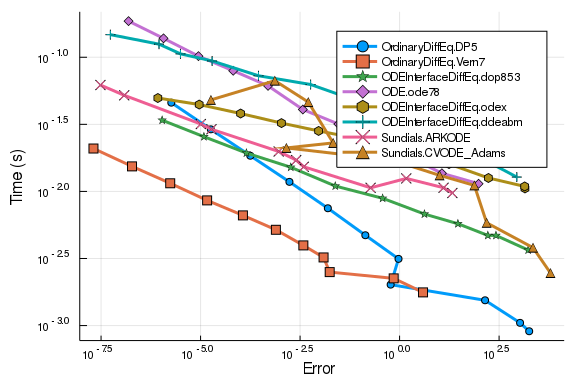Here you can once again see the DifferentialEquations algorithms far in the lead. It's well known that for cheap function costs Adams methods are inefficient. ODE.jl one again has a bad showing.

## Interpolation Error

Now we will look at the error using an interpolation measurement instead of at the timestepping points. Since the DifferentialEquations.jl algorithms have higher order interpolants than the ODE.jl algorithms, one would expect this would magnify the difference. First the order 4/5 comparison:

setups = [Dict(:alg=>DP5())
#Dict(:alg=>ode45())
Dict(:alg=>Tsit5())]
wp = WorkPrecisionSet(prob,abstols,reltols,setups;error_estimate=:L2,dense_errors=true,numruns=100)
plot(wp)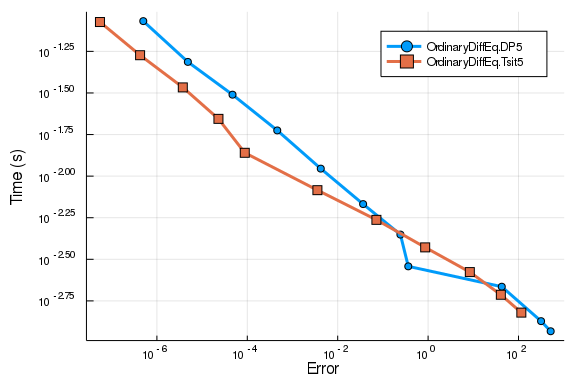Note that all of ODE.jl uses a 3rd order Hermite interpolation, while the DifferentialEquations algorithms interpolations which are specialized to the algorithm. For example, DP5 and Tsit5 both use "free" order 4 interpolations, which are both as fast as the Hermite interpolation while achieving far less error. At higher order:

setups = [Dict(:alg=>DP5())
Dict(:alg=>Vern7())
#Dict(:alg=>ode78())
]
wp = WorkPrecisionSet(prob,abstols,reltols,setups;error_estimate=:L2,dense_errors=true,numruns=100)
plot(wp)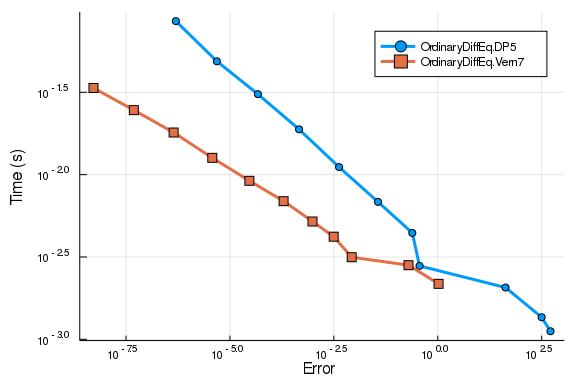## Comparison with Fixed Timestep RK4

Let's run the first benchmark but add some fixed timestep RK4 methods to see the difference:

abstols = 1.0 ./ 10.0 .^ (3:13)
reltols = 1.0 ./ 10.0 .^ (0:10);
dts = [1,1/2,1/4,1/10,1/20,1/40,1/60,1/80,1/100,1/140,1/240]
setups = [Dict(:alg=>DP5())
Dict(:alg=>ode45())
Dict(:alg=>dopri5())
Dict(:alg=>RK4(),:dts=>dts)
Dict(:alg=>Tsit5())]
solnames = ["DifferentialEquations";"ODE";"ODEInterface";"DifferentialEquations RK4";"DifferentialEquations Tsit5"]
wp = WorkPrecisionSet(prob,abstols,reltols,setups;names=solnames,
save_everystep=false,verbose=false,numruns=100)
plot(wp)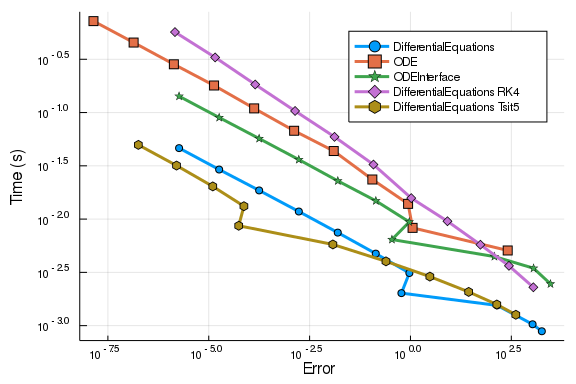## Conclusion

DifferentialEquations's default choice of Tsit5 does well for quick and easy solving at normal tolerances. However, at low tolerances the higher order algorithms are faster. In every case, the DifferentialEquations algorithms are far in the lead, many times an order of magnitude faster than the competitors. Vern7 with its included 7th order interpolation looks to be a good workhorse for scientific computing in floating point range. These along with many other benchmarks are why these algorithms were chosen as part of the defaults.

using DiffEqBenchmarks
DiffEqBenchmarks.bench_footer(WEAVE_ARGS[:folder],WEAVE_ARGS[:file])


## Appendix

These benchmarks are a part of the DiffEqBenchmarks.jl repository, found at: https://github.com/JuliaDiffEq/DiffEqBenchmarks.jl

To locally run this tutorial, do the following commands:

using DiffEqBenchmarks
DiffEqBenchmarks.weave_file("NonStiffODE","linear_wpd.jmd")

Computer Information:

Julia Version 1.1.0
Commit 80516ca202 (2019-01-21 21:24 UTC)
Platform Info:
OS: Linux (x86_64-pc-linux-gnu)
CPU: Intel(R) Xeon(R) CPU E5-2680 v4 @ 2.40GHz
WORD_SIZE: 64
LIBM: libopenlibm
LLVM: libLLVM-6.0.1 (ORCJIT, haswell)


Package Information:

Status: /home/crackauckas/.julia/environments/v1.1/Project.toml
[c52e3926-4ff0-5f6e-af25-54175e0327b1] Atom 0.8.7
[bcd4f6db-9728-5f36-b5f7-82caef46ccdb] DelayDiffEq 5.3.0
[bb2cbb15-79fc-5d1e-9bf1-8ae49c7c1650] DiffEqBenchmarks 0.1.0
[459566f4-90b8-5000-8ac3-15dfb0a30def] DiffEqCallbacks 2.5.2
[f3b72e0c-5b89-59e1-b016-84e28bfd966d] DiffEqDevTools 2.8.0
[aae7a2af-3d4f-5e19-a356-7da93b79d9d0] DiffEqFlux 0.5.0
[78ddff82-25fc-5f2b-89aa-309469cbf16f] DiffEqMonteCarlo 0.14.0
[77a26b50-5914-5dd7-bc55-306e6241c503] DiffEqNoiseProcess 3.3.1
[9fdde737-9c7f-55bf-ade8-46b3f136cc48] DiffEqOperators 3.5.0
[055956cb-9e8b-5191-98cc-73ae4a59e68a] DiffEqPhysics 3.1.0
[a077e3f3-b75c-5d7f-a0c6-6bc4c8ec64a9] DiffEqProblemLibrary 4.1.0
[41bf760c-e81c-5289-8e54-58b1f1f8abe2] DiffEqSensitivity 3.2.2
[0c46a032-eb83-5123-abaf-570d42b7fbaa] DifferentialEquations 6.4.0
[b305315f-e792-5b7a-8f41-49f472929428] Elliptic 0.5.0
[587475ba-b771-5e3f-ad9e-33799f191a9c] Flux 0.8.3
[e5e0dc1b-0480-54bc-9374-aad01c23163d] Juno 0.7.0
[7f56f5a3-f504-529b-bc02-0b1fe5e64312] LSODA 0.4.0
[c030b06c-0b6d-57c2-b091-7029874bd033] ODE 2.4.0
[54ca160b-1b9f-5127-a996-1867f4bc2a2c] ODEInterface 0.4.5
[09606e27-ecf5-54fc-bb29-004bd9f985bf] ODEInterfaceDiffEq 3.3.0
[1dea7af3-3e70-54e6-95c3-0bf5283fa5ed] OrdinaryDiffEq 5.8.1
[2dcacdae-9679-587a-88bb-8b444fb7085b] ParallelDataTransfer 0.5.0
[65888b18-ceab-5e60-b2b9-181511a3b968] ParameterizedFunctions 4.1.1
[91a5bcdd-55d7-5caf-9e0b-520d859cae80] Plots 0.25.1
[d330b81b-6aea-500a-939a-2ce795aea3ee] PyPlot 2.8.1
[731186ca-8d62-57ce-b412-fbd966d074cd] RecursiveArrayTools 0.20.0
[295af30f-e4ad-537b-8983-00126c2a3abe] Revise 2.1.6
[90137ffa-7385-5640-81b9-e52037218182] StaticArrays 0.11.0
[789caeaf-c7a9-5a7d-9973-96adeb23e2a0] StochasticDiffEq 6.2.0
[c3572dad-4567-51f8-b174-8c6c989267f4] Sundials 3.6.0
[92b13dbe-c966-51a2-8445-caca9f8a7d42] TaylorIntegration 0.5.0
[44d3d7a6-8a23-5bf8-98c5-b353f8df5ec9] Weave 0.9.0
[e88e6eb3-aa80-5325-afca-941959d7151f] Zygote 0.3.1